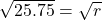## The area of a circle is 80.86cm2. Find the length of the radius rounded to 2 DP.

Question

The area of a circle is 80.86cm2.
Find the length of the radius rounded to 2 DP.

in progress 0
6 months 2021-07-21T01:46:19+00:00 1 Answers 4 views 0

Solution given:

The area of a circle =80.86cm²

we have:

The area of a circle =πr²

substituting value of area of circle we get

80.86cm=3.14*r²

dividing both side by 3.14

80.86/3.14=3.14*r²/3.14

25.75=r²

doing square root on both sider=5.07cm

The length of radius is 5.07cm